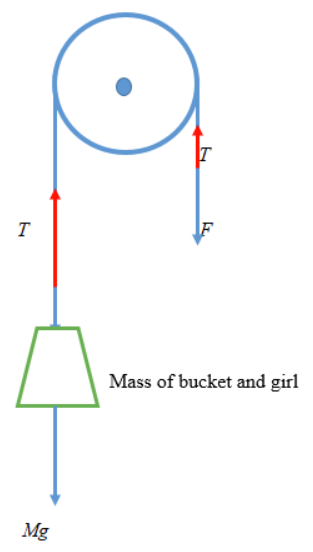# Problem: A window washer pulls herself upward using the bucket-pulley apparatus shown in the figure.The mass of the person plus the bucket is 61 kg. (a) How hard must she pull downward to raise herself slowly at constant speed? (b) If she increases this force by 19%, what will her acceleration be?

###### FREE Expert Solution

Newton's second law:

$\overline{){\mathbf{\Sigma }}{\mathbf{F}}{\mathbf{=}}{\mathbf{m}}{\mathbf{a}}}$

(a)

At constant speed, acceleration is zero.

The force applied by the girl is not an external force.

We have two "T" pulling upward and Mg acting downward.

ΣF = T + T - Mg = 0

2T = Mg

95% (290 ratings)###### Problem Details

A window washer pulls herself upward using the bucket-pulley apparatus shown in the figure.The mass of the person plus the bucket is 61 kg.

(a) How hard must she pull downward to raise herself slowly at constant speed?

(b) If she increases this force by 19%, what will her acceleration be?